# List Comprehension Review Lists Loops Lists Loops fruit

• Slides: 32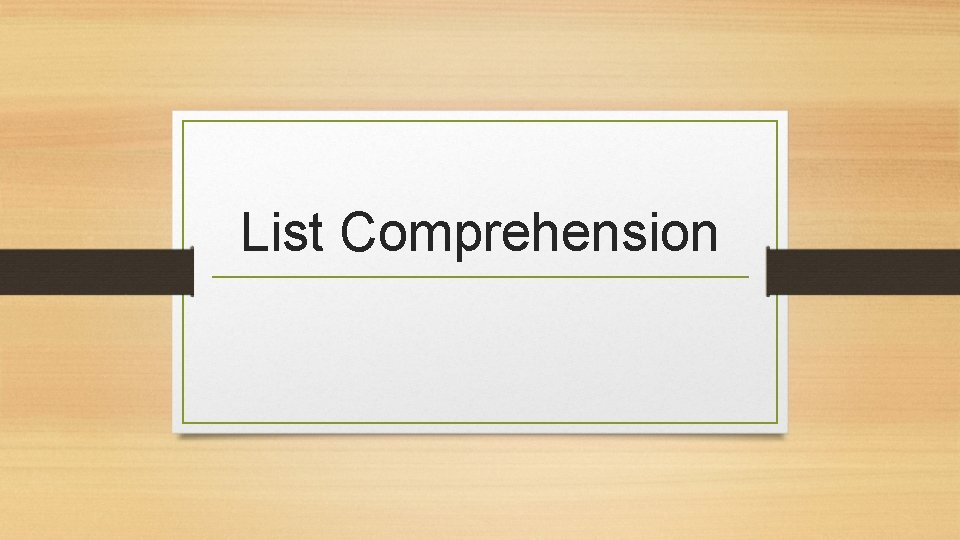List ComprehensionReview Lists & Loops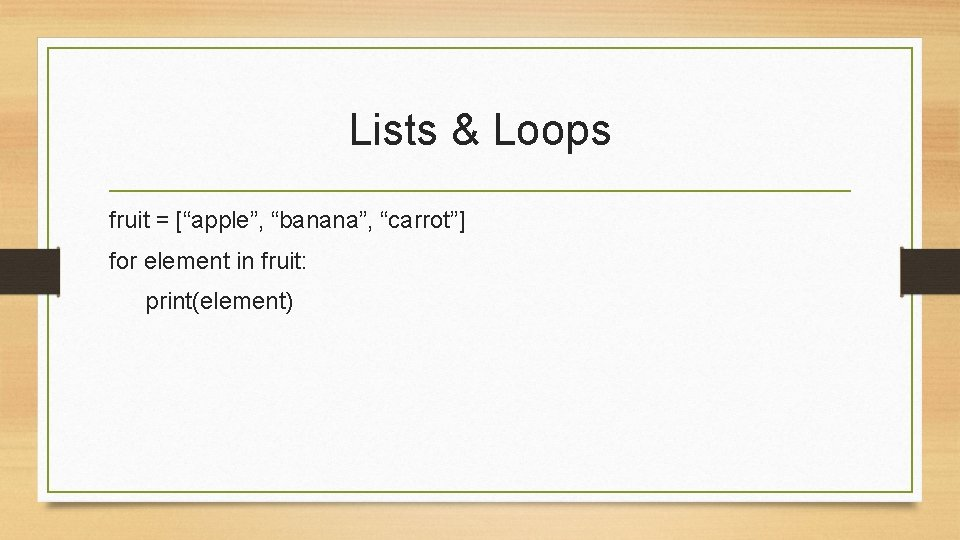Lists & Loops fruit = [“apple”, “banana”, “carrot”] for element in fruit: print(element)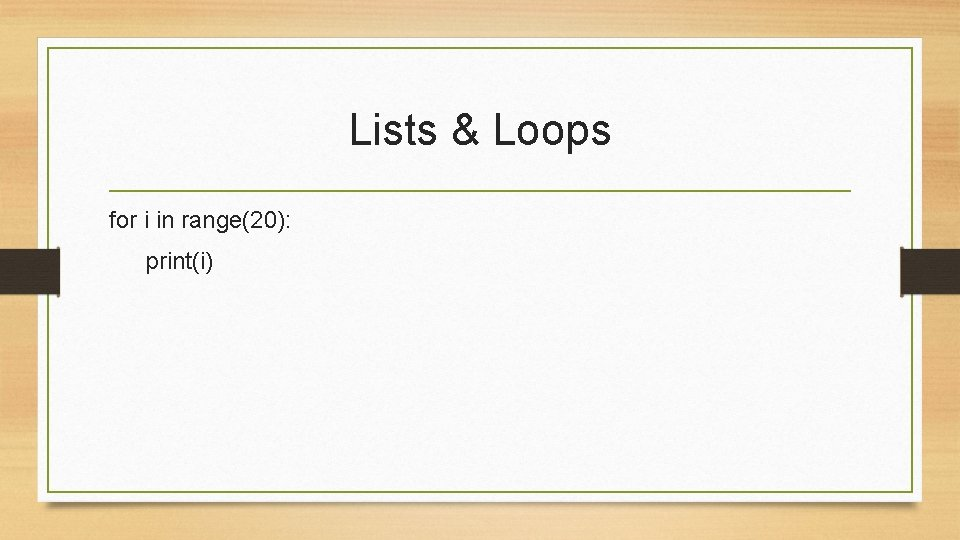Lists & Loops for i in range(20): print(i)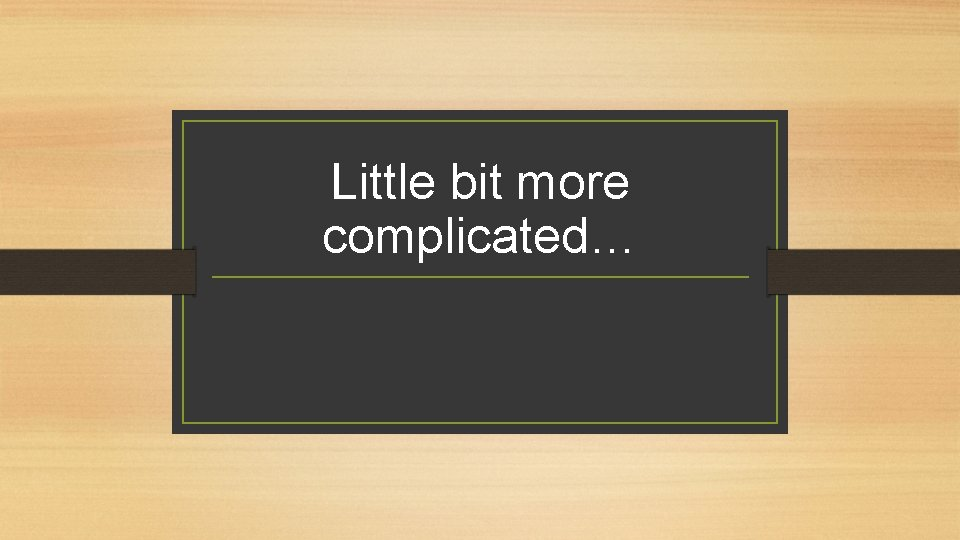Little bit more complicated…Goal: [“apples”, “bananas”, “carrots”] fruit = [“apple”, “banana”, “carrot”] for element in fruit: print(element + “s”) BUT THAT DOESN’T SAVE MY NEW LISTGoal: [“apples”, “bananas”, “carrots”] fruit = [“apple”, “banana”, “carrot”] morefruit = [ ] for element in fruit: new = element + “s” morefruit. append(new) print(morefruit) 1. Start with an empty list 2. Create a new object in loop 3. Append new object to new list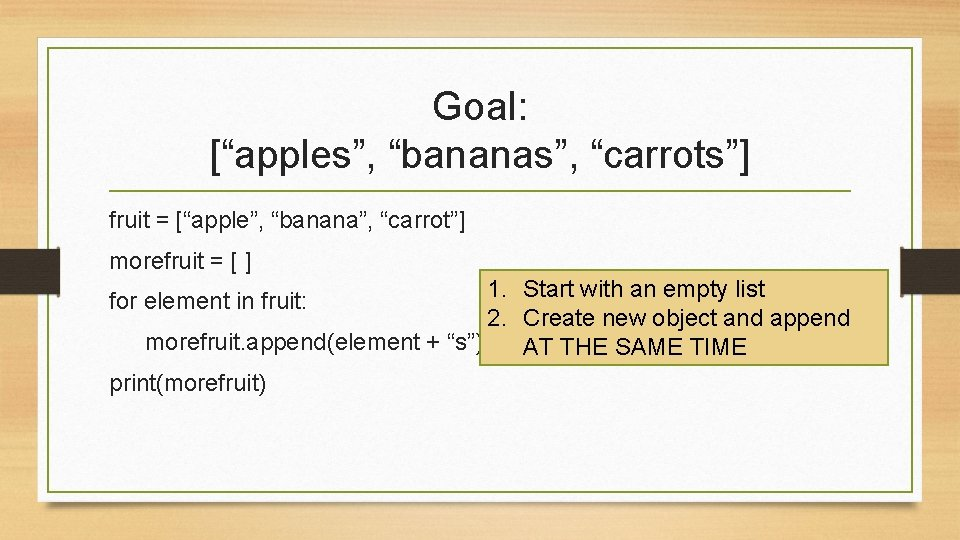Goal: [“apples”, “bananas”, “carrots”] fruit = [“apple”, “banana”, “carrot”] morefruit = [ ] 1. Start with an empty list 2. Create new object and append morefruit. append(element + “s”) AT THE SAME TIME print(morefruit) for element in fruit: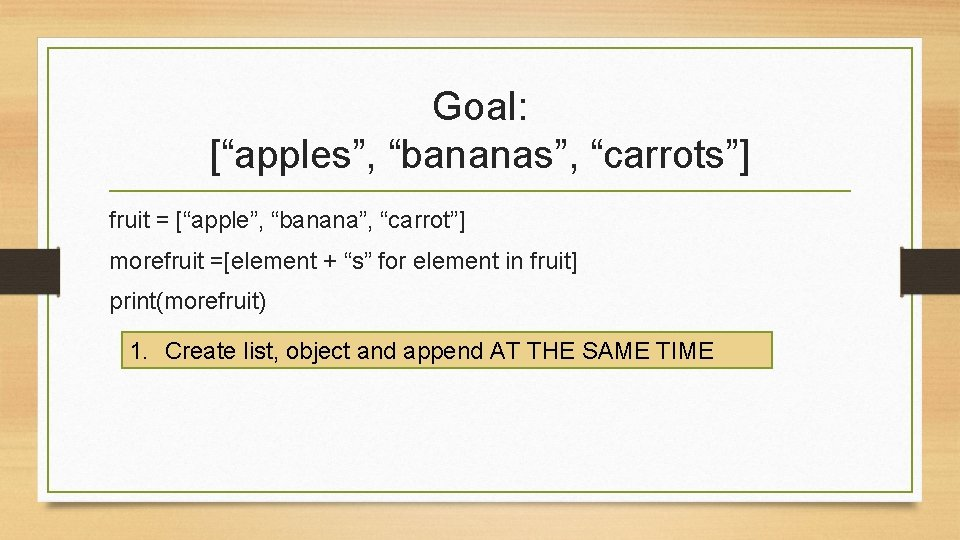Goal: [“apples”, “bananas”, “carrots”] fruit = [“apple”, “banana”, “carrot”] morefruit =[element + “s” for element in fruit] print(morefruit) 1. Create list, object and append AT THE SAME TIME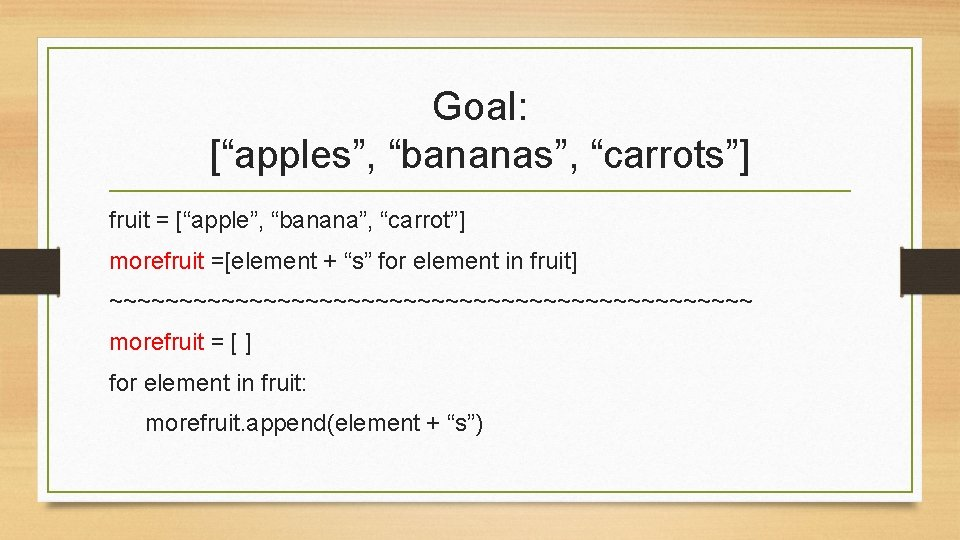Goal: [“apples”, “bananas”, “carrots”] fruit = [“apple”, “banana”, “carrot”] morefruit =[element + “s” for element in fruit] ~~~~~~~~~~~~~~~~~~~~~~~ morefruit = [ ] for element in fruit: morefruit. append(element + “s”)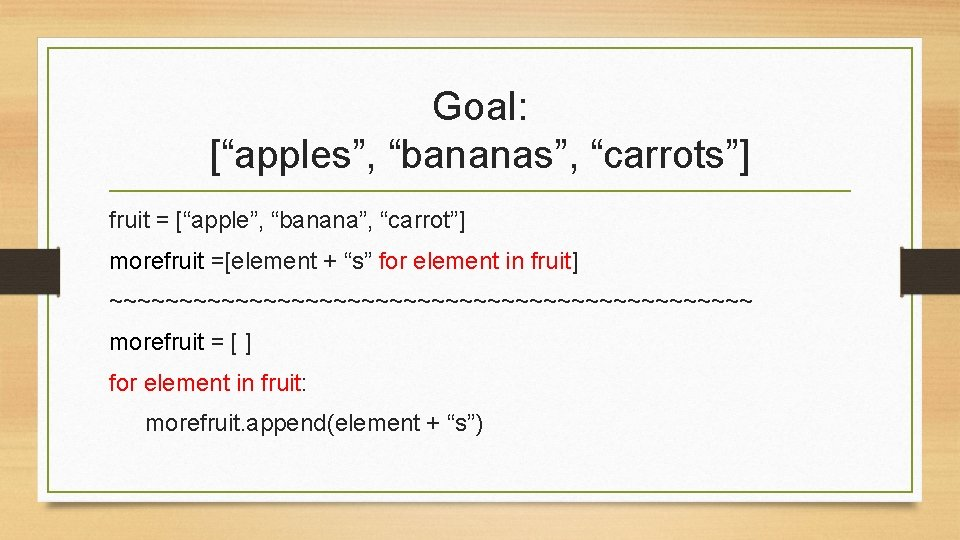Goal: [“apples”, “bananas”, “carrots”] fruit = [“apple”, “banana”, “carrot”] morefruit =[element + “s” for element in fruit] ~~~~~~~~~~~~~~~~~~~~~~~ morefruit = [ ] for element in fruit: morefruit. append(element + “s”)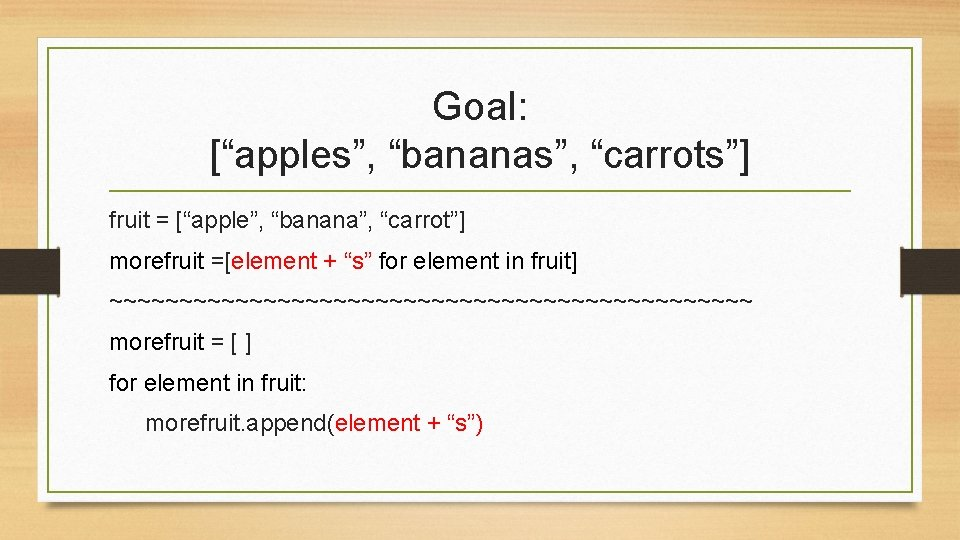Goal: [“apples”, “bananas”, “carrots”] fruit = [“apple”, “banana”, “carrot”] morefruit =[element + “s” for element in fruit] ~~~~~~~~~~~~~~~~~~~~~~~ morefruit = [ ] for element in fruit: morefruit. append(element + “s”)Goal: [1, 4, 9, 16, 25, 36, 49, 64, 81, 100] squares = [ ] for num in range(1, 11): new = num ** 2 squares. append(new) print(squares) 1. Start with an empty list 2. Create a new object in loop 3. Append new object to new list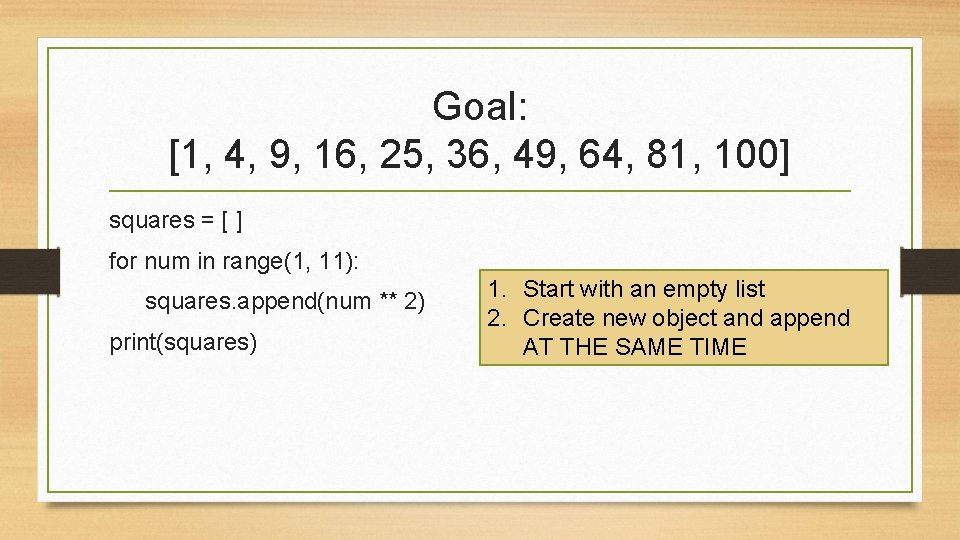Goal: [1, 4, 9, 16, 25, 36, 49, 64, 81, 100] squares = [ ] for num in range(1, 11): squares. append(num ** 2) print(squares) 1. Start with an empty list 2. Create new object and append AT THE SAME TIME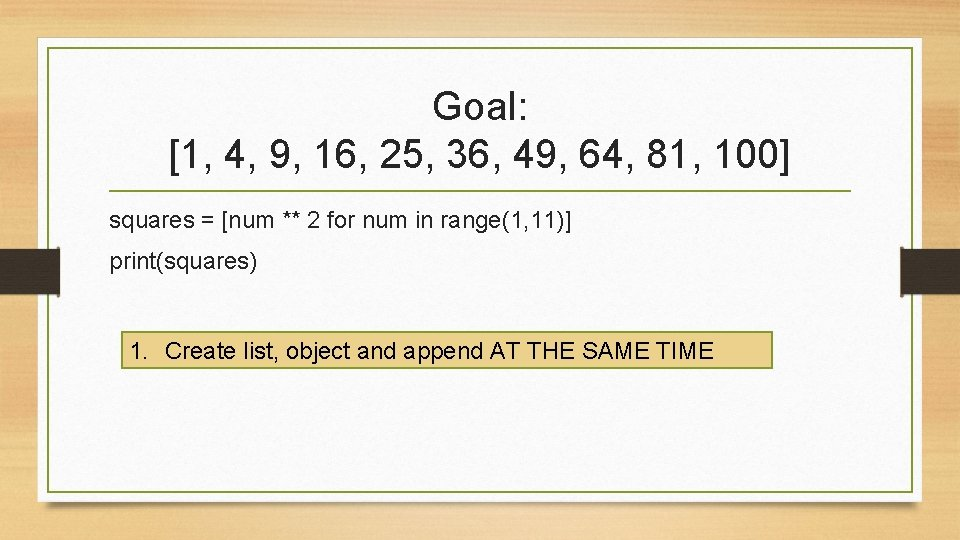Goal: [1, 4, 9, 16, 25, 36, 49, 64, 81, 100] squares = [num ** 2 for num in range(1, 11)] print(squares) 1. Create list, object and append AT THE SAME TIMEGoal: [1, 4, 9, 16, 25, 36, 49, 64, 81, 100] squares = [num ** 2 for num in range(1, 11)] print(squares) ~~~~~~~~~~~~~~~~~~~~ squares = [ ] for num in range(1, 11): squares. append(num ** 2)Goal: [1, 4, 9, 16, 25, 36, 49, 64, 81, 100] squares = [num ** 2 for num in range(1, 11)] print(squares) ~~~~~~~~~~~~~~~~~~~~ squares = [ ] for num in range(1, 11): squares. append(num ** 2)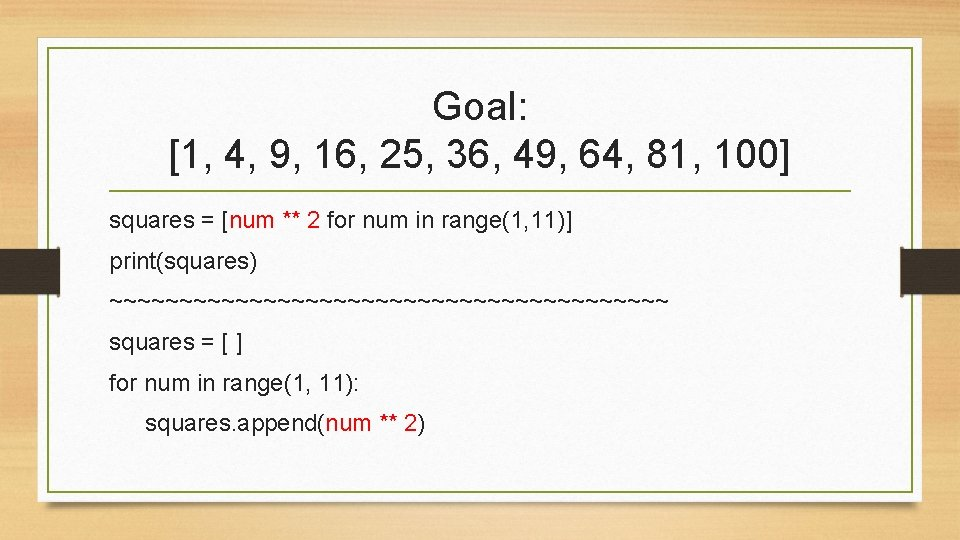Goal: [1, 4, 9, 16, 25, 36, 49, 64, 81, 100] squares = [num ** 2 for num in range(1, 11)] print(squares) ~~~~~~~~~~~~~~~~~~~~ squares = [ ] for num in range(1, 11): squares. append(num ** 2)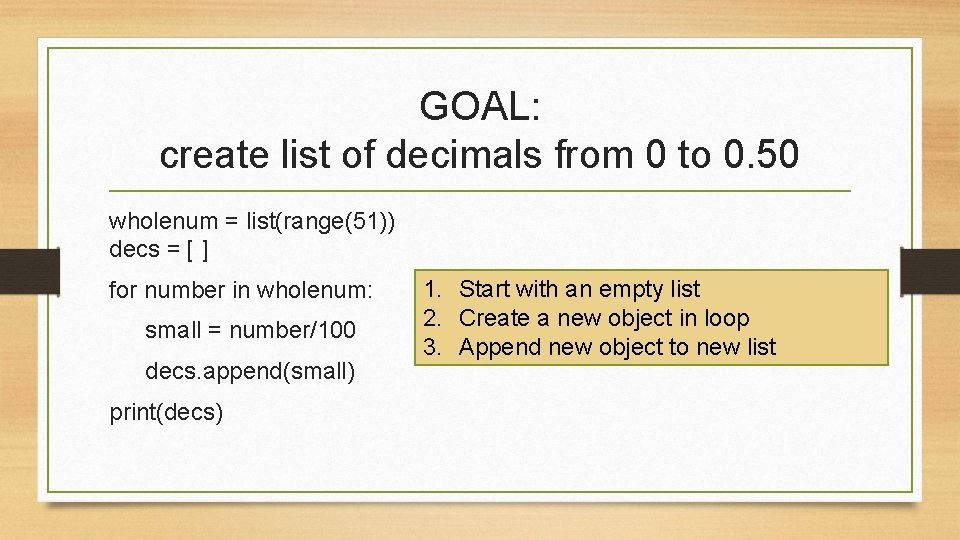GOAL: create list of decimals from 0 to 0. 50 wholenum = list(range(51)) decs = [ ] for number in wholenum: small = number/100 decs. append(small) print(decs) 1. Start with an empty list 2. Create a new object in loop 3. Append new object to new list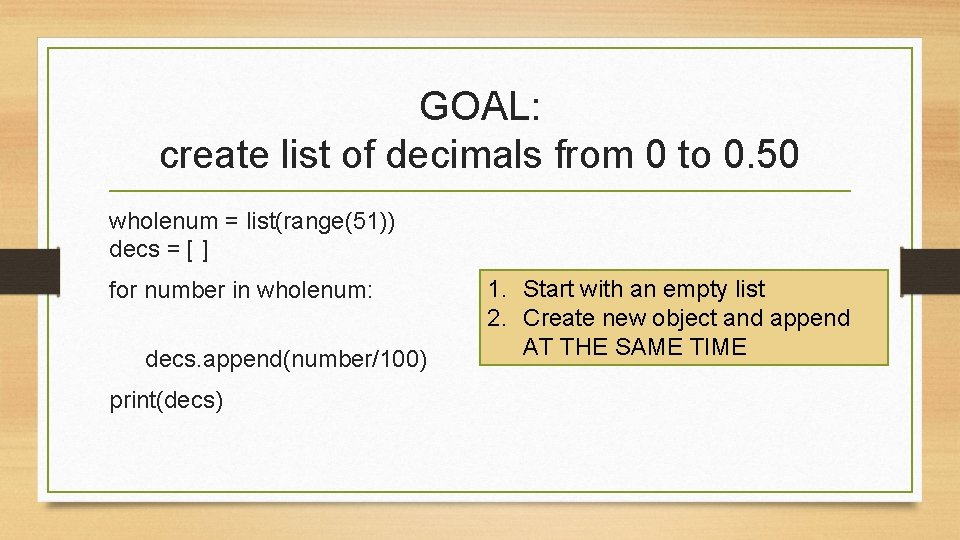GOAL: create list of decimals from 0 to 0. 50 wholenum = list(range(51)) decs = [ ] for number in wholenum: decs. append(number/100) print(decs) 1. Start with an empty list 2. Create new object and append AT THE SAME TIME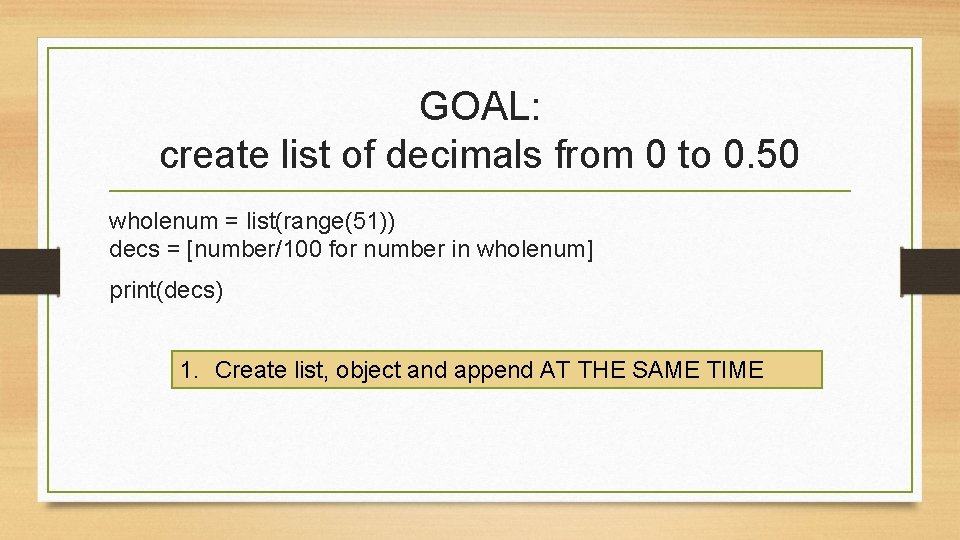GOAL: create list of decimals from 0 to 0. 50 wholenum = list(range(51)) decs = [number/100 for number in wholenum] print(decs) 1. Create list, object and append AT THE SAME TIMEGOAL: create list of decimals from 0 to 0. 50 wholenum = list(range(51)) decs = [number/100 for number in wholenum] print(decs) ~~~~~~~~~~~~~~~~~~~ decs = [ ] for number in wholenum: decs. append(number/100)GOAL: create list of decimals from 0 to 0. 50 wholenum = list(range(51)) decs = [number/100 for number in wholenum] print(decs) ~~~~~~~~~~~~~~~~~~~ decs = [ ] for number in wholenum: decs. append(number/100)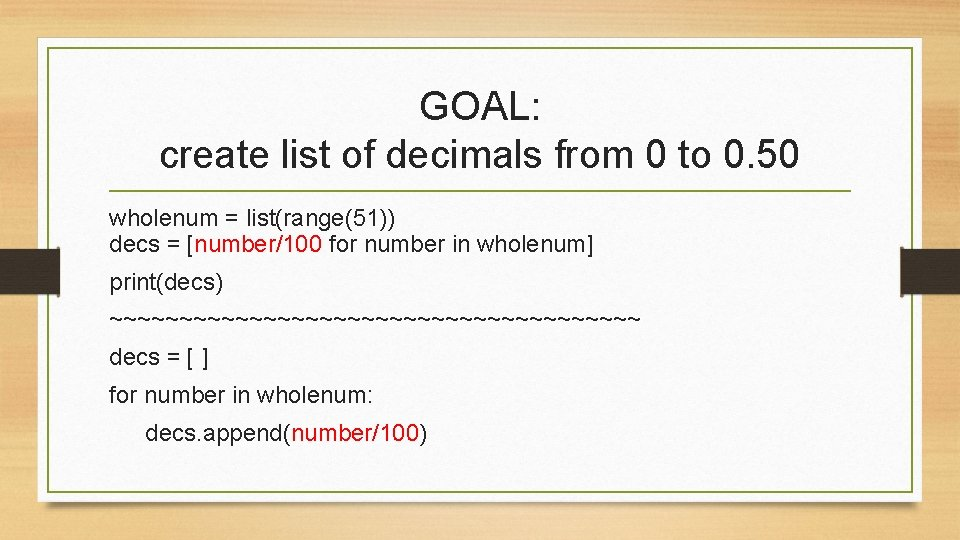GOAL: create list of decimals from 0 to 0. 50 wholenum = list(range(51)) decs = [number/100 for number in wholenum] print(decs) ~~~~~~~~~~~~~~~~~~~ decs = [ ] for number in wholenum: decs. append(number/100)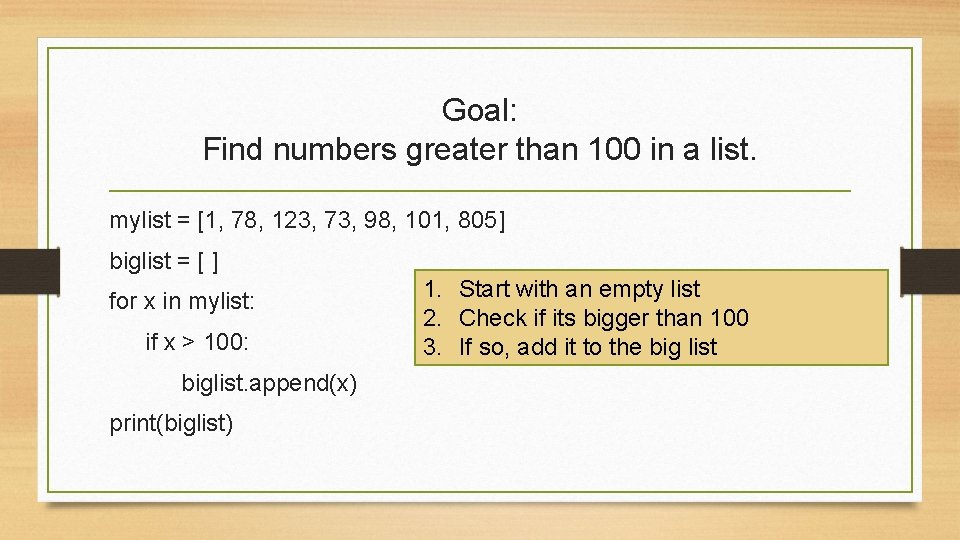Goal: Find numbers greater than 100 in a list. mylist = [1, 78, 123, 73, 98, 101, 805] biglist = [ ] for x in mylist: if x > 100: biglist. append(x) print(biglist) 1. Start with an empty list 2. Check if its bigger than 100 3. If so, add it to the big list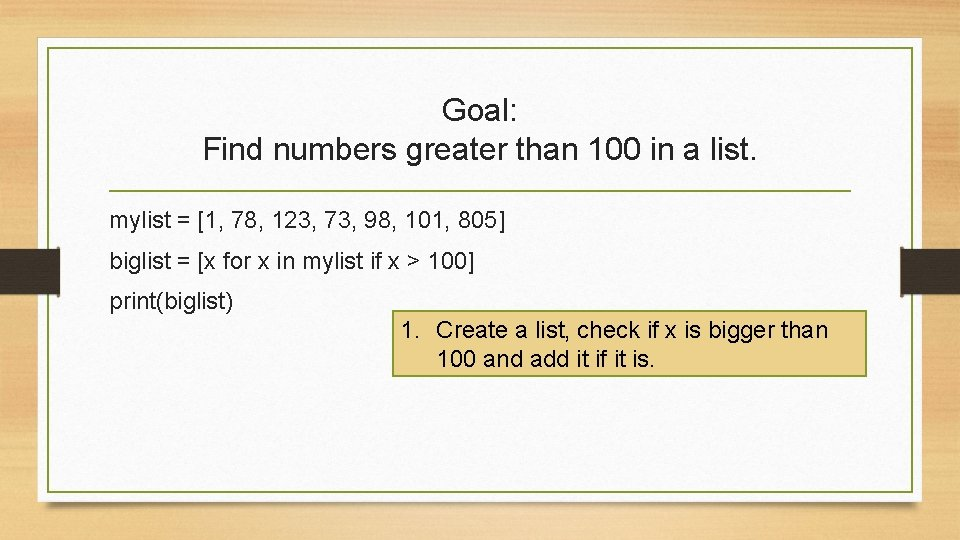Goal: Find numbers greater than 100 in a list. mylist = [1, 78, 123, 73, 98, 101, 805] biglist = [x for x in mylist if x > 100] print(biglist) 1. Create a list, check if x is bigger than 100 and add it if it is.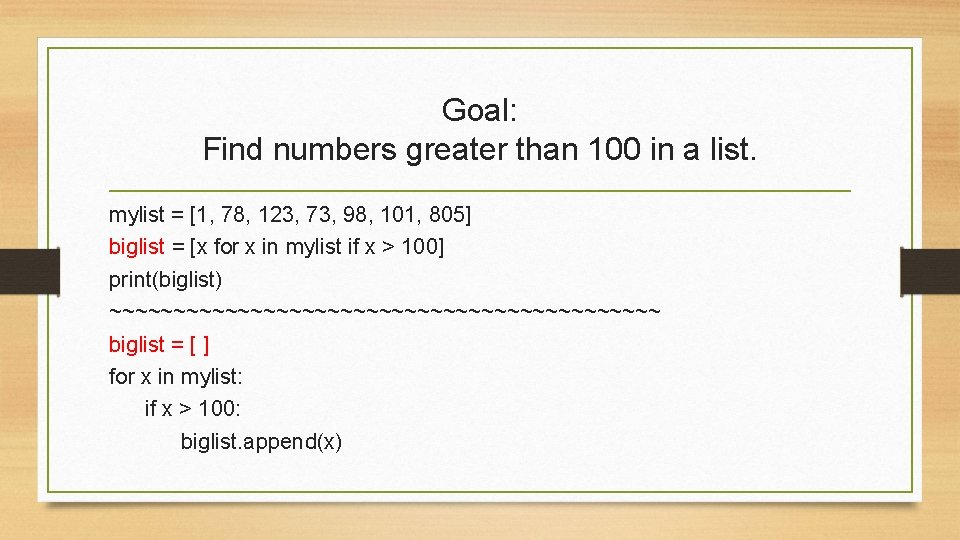Goal: Find numbers greater than 100 in a list. mylist = [1, 78, 123, 73, 98, 101, 805] biglist = [x for x in mylist if x > 100] print(biglist) ~~~~~~~~~~~~~~~~~~~~~~ biglist = [ ] for x in mylist: if x > 100: biglist. append(x)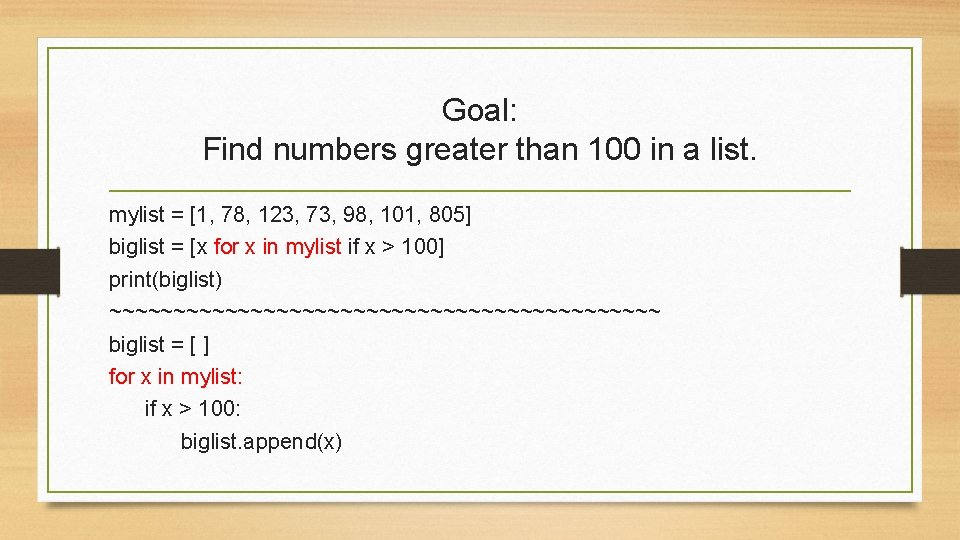Goal: Find numbers greater than 100 in a list. mylist = [1, 78, 123, 73, 98, 101, 805] biglist = [x for x in mylist if x > 100] print(biglist) ~~~~~~~~~~~~~~~~~~~~~~ biglist = [ ] for x in mylist: if x > 100: biglist. append(x)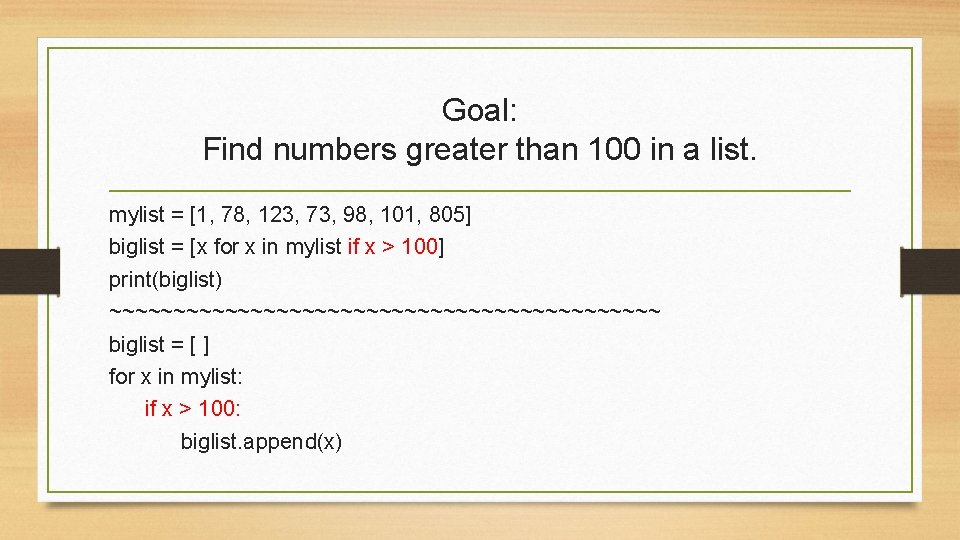Goal: Find numbers greater than 100 in a list. mylist = [1, 78, 123, 73, 98, 101, 805] biglist = [x for x in mylist if x > 100] print(biglist) ~~~~~~~~~~~~~~~~~~~~~~ biglist = [ ] for x in mylist: if x > 100: biglist. append(x)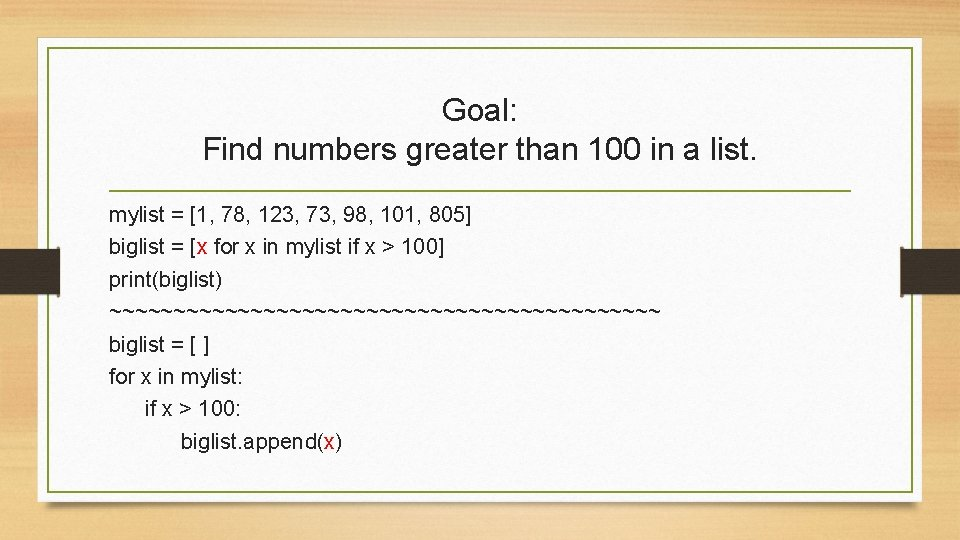Goal: Find numbers greater than 100 in a list. mylist = [1, 78, 123, 73, 98, 101, 805] biglist = [x for x in mylist if x > 100] print(biglist) ~~~~~~~~~~~~~~~~~~~~~~ biglist = [ ] for x in mylist: if x > 100: biglist. append(x)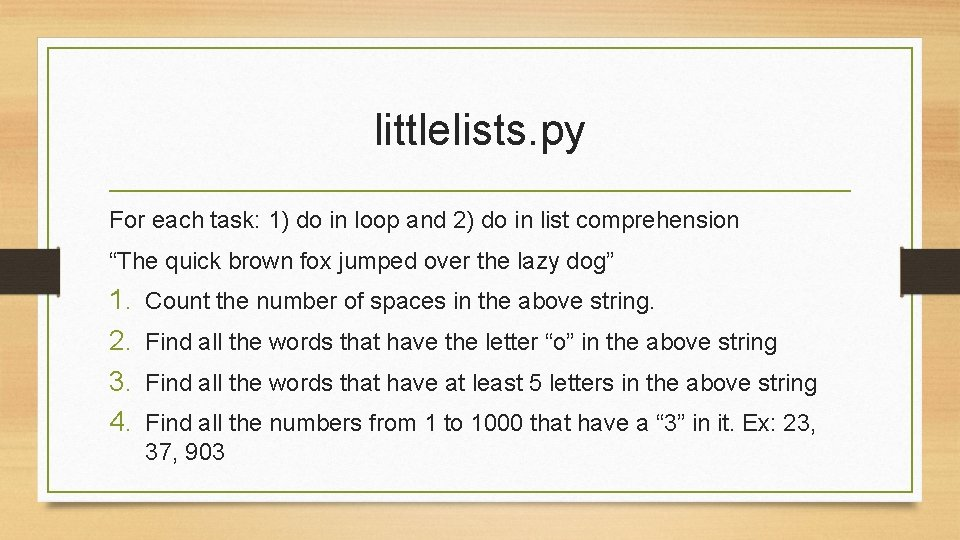littlelists. py For each task: 1) do in loop and 2) do in list comprehension “The quick brown fox jumped over the lazy dog” 1. 2. 3. 4. Count the number of spaces in the above string. Find all the words that have the letter “o” in the above string Find all the words that have at least 5 letters in the above string Find all the numbers from 1 to 1000 that have a “ 3” in it. Ex: 23, 37, 903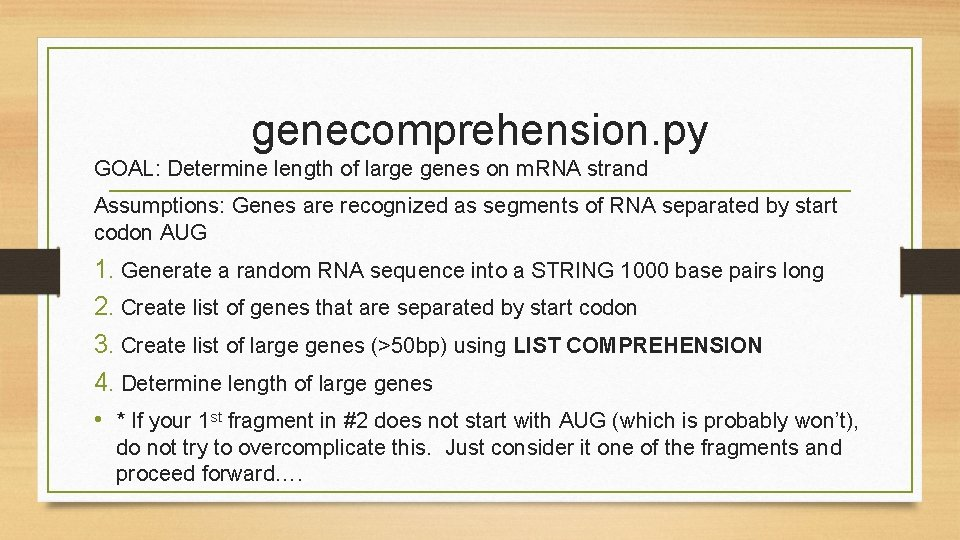genecomprehension. py GOAL: Determine length of large genes on m. RNA strand Assumptions: Genes are recognized as segments of RNA separated by start codon AUG 1. Generate a random RNA sequence into a STRING 1000 base pairs long 2. Create list of genes that are separated by start codon 3. Create list of large genes (>50 bp) using LIST COMPREHENSION 4. Determine length of large genes • * If your 1 st fragment in #2 does not start with AUG (which is probably won’t), do not try to overcomplicate this. Just consider it one of the fragments and proceed forward….